Updating search results...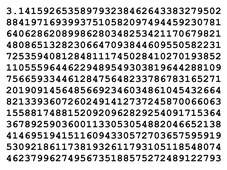# Mathematics

Algebra, Calculus, Functions, Geometry, Numbers and Operations, Measurement and Data, Ratios and Proportions, Statistics and Probability, Trigonometry.

14 affiliated resources

# Search Resources

View
Selected filters:Unrestricted Use
CC BY
Rating
0.0 stars

Topics include signed numbers, decimal numbers, exponential notation, scientific notation, solving and graphing linear equations, an introduction to polynomials, and systems of linear equations and their graphs. Geometrical topics include lines and angles, closed curves and convex polygons, triangles and similarities, and symmetry and proportion in nature and art. Students may complete this course during the first three weeks of the semester by passing the MyMathLab modules. Students will then be eligible to take either MAT 099 Intermediate Algebra, MAT 114-Quantitative Reasoning or MAT 120-Intro to Statistics the following semester. This course does not satisfy degree requirements. Students may complete this course during the first three weeks of the semester by passing the MyOpenMath Acceleration assignments.

Subject:
Mathematics
Material Type:
Full Course
Provider:
Roxbury Community College
Author:
Valerie Dietel-Brenneman
05/15/2019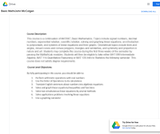Unrestricted Use
CC BY
Rating
0.0 stars

This course is a continuation of MAT087, Basic Mathematics. Topics include signed numbers, decimal numbers, exponential notation, scientific notation, solving and graphing linear equations, an introduction to polynomials, and systems of linear equations and their graphs. Geometrical topics include lines and angles, closed curves and convex polygons, triangles and similarities, and symmetry and proportion in nature and art. Students may complete this course during the first three weeks of the semester by passing the MyMathLab modules. Students will then be eligible to take either MAT 099 Intermediate Algebra, MAT 114-Quantitative Reasoning or MAT 120-Intro to Statistics the following semester. This course does not satisfy degree requirements.

Subject:
Mathematics
Material Type:
Full Course
Provider:
Roxbury Community College
Author:
John McColgan
05/15/2019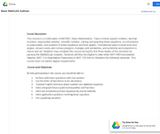Unrestricted Use
CC BY
Rating
0.0 stars

This course is a continuation of MAT087, Basic Mathematics. Topics include signed numbers, decimal numbers, exponential notation, scientific notation, solving and graphing linear equations, an introduction to polynomials, and systems of linear equations and their graphs. Geometrical topics include lines and angles, closed curves and convex polygons, triangles and similarities, and symmetry and proportion in nature and art. Students may complete this course during the first three weeks of the semester by passing the MyMathLab modules. Students will then be eligible to take either MAT 099 Intermediate Algebra, MAT 114-Quantitative Reasoning or MAT 120-Intro to Statistics the following semester. This course does not satisfy degree requirements.

Subject:
Mathematics
Material Type:
Full Course
Provider:
Northern Essex Community College
Author:
Jim Sullivan
05/15/2019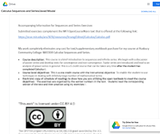Unrestricted Use
CC BY
Rating
0.0 stars

This course is a brief introduction to sequences and infinite series. We begin with a discussion of power series and develop tests for convergence and non-convergence. Taylor series are introduced and lead to an analysis of power series in general. This is a 1-credit course that can be taken any time after the student has completed Calculus I.

Subject:
Calculus
Material Type:
Full Course
Provider:
Roxbury Community College
Author:
05/15/2019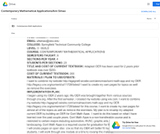Unrestricted Use
CC BY
Rating
0.0 stars

Full course on Contemporary Mathematical Applications.

Subject:
Mathematics
Material Type:
Full Course
Provider:
Springfield Technical Community College
Author:
Ann Simao
05/15/2019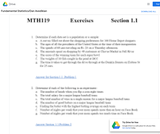Unrestricted Use
CC BY
Rating
0.0 stars

Introductory Statistics Course covering hypothesis testing, confidence interval, sampling, probability, counting techniques, correlation, linear regression, data collection and more.

Subject:
Statistics and Probability
Material Type:
Full Course
Provider:
Bristol Community College
Author:
Dan Avedikian
05/01/2019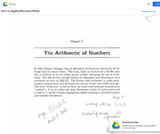Unrestricted Use
CC BY
Rating
0.0 stars

Introductory Algebra is the second of three classes in the developmental mathematics sequence. It provides a development of concepts of variables, expressions, and equations using symbolic algebra to represent primarily linear relationships both graphically and analytically. The concept of function will be developed for the application of linear equations and concepts of dependent and independent variable. Students will also learn to solve simultaneous linear equations as well as how to construct linear equations from slope and point information. Application problems will include geometric figure quantities, ratio and proportion, direct and indirect variation, and conversion of units. Finding the greatest common factor of a polynomial will also be included. The emphasis of the three semester sequence is fortification of mental calculation power with minimum reliance on digital calculation.

Subject:
Algebra
Material Type:
Full Course
Provider:
MassBay Community College
Author:
Ravindra Bhalla
05/13/2019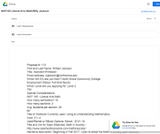Unrestricted Use
CC BY
Rating
0.0 stars

A course consisting of mathematical topics chosen to provide an overview of a broad range

of higher mathematics to aid students in interpreting an understanding current issues and

to increase students’ ability to reason and think critically. Topics may include but are not

limited to reasoning and problem solving, sets, logic, social choice, numeration systems,

growth and symmetry, and fractals.

Subject:
Mathematics
Material Type:
Full Course
Provider:
North Shore Community College
Author:
William Jackson
05/14/2019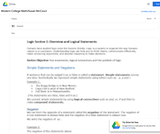Unrestricted Use
CC BY
Rating
0.0 stars

Survey course for college mathematics.

Subject:
Mathematics
Material Type:
Full Course
Provider:
Bristol Community College
Author:
Susan McCourt
05/01/2019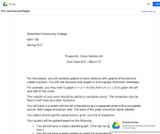Unrestricted Use
CC BY
Rating
0.0 stars

A preparation for MAT 201 and the physical sciences. Students study principles, properties, and

applications of functions and their graphs. They revisit functions introduced in MAT 107 and expand

their knowledge of them. The course focuses heavily on circular trigonometric functions and introduces

conic sections. Laboratory activities place particular emphasis on the use of functions to model real

problems. Computers and/or graphing calculators are used to enhance the study of mathematics.

Prerequisites: A grade of C- or better in MAT 107 or a score of 71 or higher on the College Level Math

placement test; ENG 090 and ENG 094 (COL 090), or satisfactory placement test scores.

Subject:
Calculus
Material Type:
Full Course
Provider:
Greenfield Community College
Author:
Jodi Nager
01/01/2017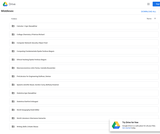Unrestricted Use
CC BY
Rating
0.0 stars

The goals of this course are to give students a greater knowledge and understanding of mathematics and to help students develop new skills and concepts and enhance their problem-solving ability, all of which are necessary for the study of a science and engineering oriented calculus. MAT 196 is also designed to help students further develop and extend their critical thinking skills in a contextualized environment. You will achieve this goal by applying strategies presented by the instructor which are designed to help you interpret, analyze, evaluate, infer, and synthesize concepts studied in preparation for Calculus.

Subject:
Calculus
Material Type:
Full Course
Provider:
Middlesex Community College
Author:
Denise Sullivan
05/13/2019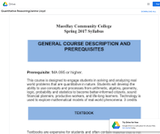Unrestricted Use
CC BY
Rating
0.0 stars

This course is designed to engage students in solving and analyzing real world problems that are quantitative in nature. Students will develop the ability to use concepts and processes from arithmetic, algebra, geometry, logic, probability and statistics to become better-informed citizens, sound financial planners, productive workers, and life-long learners. Technology is used to explore mathematical models of real-world phenomena. 3 credits

Subject:
Mathematics
Material Type:
Full Course
Provider:
MassBay Community College
Author:
Janine Lloyd
05/08/2019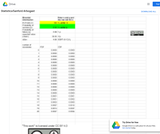Unrestricted Use
CC BY
Rating
0.0 stars

A general statistics course, which includes understanding data, measures of central tendency, measures of variation, binomial distributions, normal distributions, correlation and regression, probability and sampling distributions, Central Limit Theorem, confidence intervals, estimates of population parameters and hypothesis testing. Interpretation and data analysis are emphasized.

Subject:
Statistics and Probability
Material Type:
Full Course
Provider:
Middlesex Community College
Author:
Sanford Arbogast
05/13/2019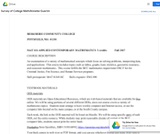Unrestricted Use
CC BY
Rating
0.0 stars

An examination of a variety of mathematical concepts which focus on solving problems, interpreting data, and applications. This course includes topics such as tables, graphs, basic statistics, geometric measures, and consumer mathematics. This course fulfills the BCC mathematics requirement ONLY for the Criminal Justice, Fire Science, and Human Services programs.

Subject:
Mathematics
Material Type:
Full Course
Provider:
Berkshire Community College
Author:
Annette Guertin